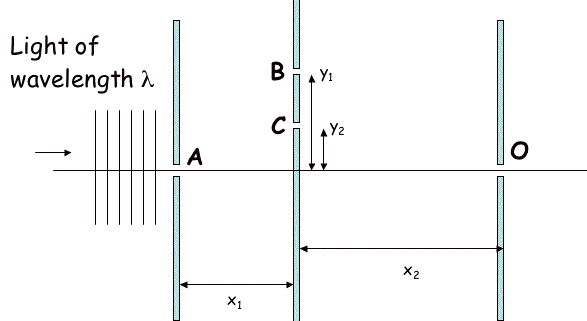# Two Offset Slits

• Gee Wiz
In summary, the conversation discusses a setup with three screens and multiple slits for light to pass through. The light intensity at a specific point is measured when different combinations of slits are open. The conversation also mentions the use of equations such as the law of cosine and the Pythagorean theorem to calculate the phase difference between the light passing through different slits. After some discussion and calculations, the correct value for the light intensity is determined to be 0.2563 W/m2.

## Homework Statement

Consider the above setup, not drawn to scale.

Light of wavelength λ= 475 nm strikes the first screen with slit A.
The second screen, x1 = 0.7 meters behind the first screen, has two slits, B and C .
The third screen is x2 = 1.5 meters behind the second screen. It has slit O, which is level with slit A. A lightmeter measures the light intensity at the slit O.

When light is sent through slit A and measured at the slit O with either slit B or slit C open one slit at a time, the intensity at the point O is the same: I0 = 0.5 W/m2. (The slit widths can always be adjusted so that this is true, but for this problem you can/should ignore the width of all slits.)

Slit B is at height y1 = 2 mm above slit A.

Slit C is at height y2 = 1 mm above slit A.

Note that the drawing is not drawn to scale.

1) What is the light intensity measured at the point O when both slits B and C are open?
I =?## Homework Equations

sin(theta)=(n*lamda)/(d)
Law of cosine

## The Attempt at a Solution

I believe that the light enters B and C is out of phase. I tried to find delta using the Pythagorean theorem and got 3.14e-6. Then I used 2*pi*delta/lamba to get what i thought would be phi. Then i did 4*.5*cos^2(phi/2)...but that didn't work. I got something around .256 but that was incorrect

Last edited:
I think you have the right approach. I'm not getting the same value for the the path difference between path ABO and path ACO. {EDIT: Nevermind! I agree with your value for delta.}

Last edited:

I tried that as well, so only quote unquote hint I get is :
"Note that the waves coming to slits B and C are NOT necessarily in phase with each other. You can either take this into account explicitly, or note that all rays that pass through O also pass through A, and the upper-path and lower-path rays ARE in phase at A. You will need to carry out your calculations carefully, keeping many decimal points. How many? The answer needs to be good to within 1%. How accurately does phi need to be specified? And how accurately then does the path length difference need to be specified, and how many decimal places out of the total path length is this? Make sure you keep track of units!"

I have not been rounding anything in my calculations. My calculator gives me about 10 spots/places after the decimal place...

Oh wait, I just got an email from my professor saying the system is having issues with presicion...so i guess i'll wait a little while until it is back up to continue (maybe i actually have the right answer)

OK. Now I'm getting .256 in agreement with you. Good.

So apparently the .256 is not correct, but one of the random answers i put earlier .23305 is correct. Although I'm not sure how i got that.

Interesting. I don't see why your answer isn't correct. I'll try to get some of the other helpers around here to look at it.

With a taylor approximation to second and third order, I can confirm .25627 W.
Using only first order, I get 0.25632 W. To get a wrong answer of .23305, you need some significant rounding errors.

Thank you guys so much. It turns out that .2563 is correct (there was just apparently a lot of issues with the online system)